SSC CGL Previous Year Question Paper 1 September 2016: Gener - Study24x7Default error msg

New to Study24x7 ?

# SSC CGL Previous Year Question Paper 1 September 2016: General Intelligence & Reasoning

Updated on 25 March 2020SSC Preparation Strategies & S
Updated on 25 March 2020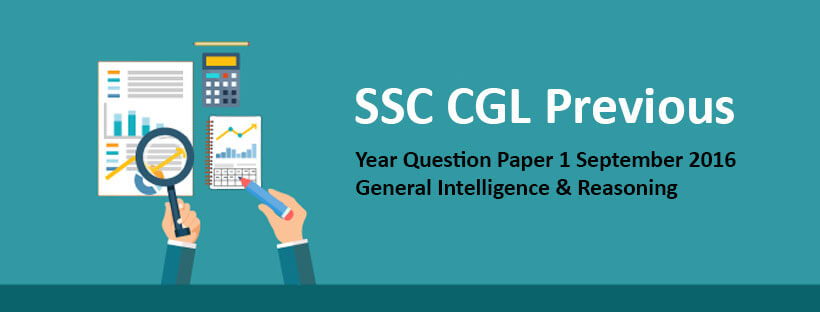1. Select the related word/letters/number from the given alternatives.

Psychology : Human Being :: Ornithology : ?

a. Birds

b. Volcanoes

c. Insects

d. Reptiles

2. Select the related word/letters/number from the given alternatives.

EGIK : FHJL :: MOQS : ?

a. LNOQ

b. NPRT

c. KMOQ

d. NRPT

3. Select the related word/letters/number from the given alternatives.

147 : 741 :: 869 : ?

a. 896

b. 968

c. 689

d. 986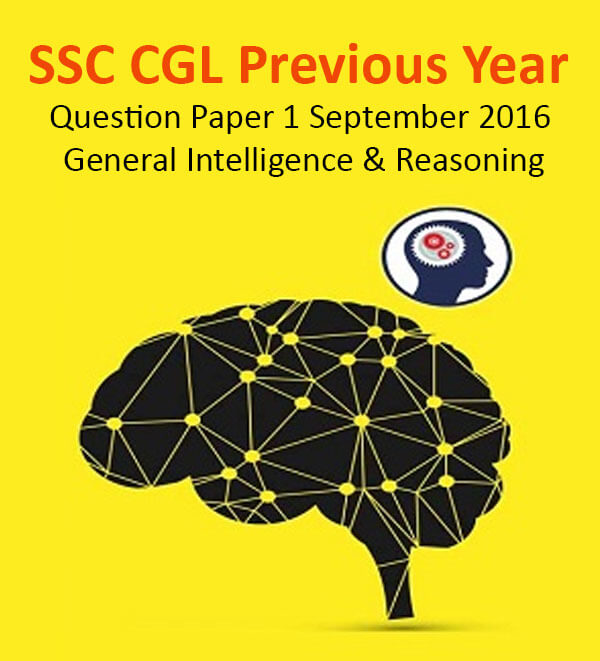4. Find the odd word/letters/number pair from the given alternatives.

a. Herb

b. Flower

c. Tree

d. Shrub

5. Find the odd word/letters/number pair from the given alternatives.

a. JKLM

b. NOPQ

c. RSTU

d. VWXZ

SSC CGL tier 1 Exam : preparation strategy

6. Find the odd word/letters/number pair from the given alternatives.

a. (85, 136)

b. (34, 85)

c. (102, 153)

d. (63, 162)

7. Arrange the following words as per order in the dictionary

1. Organ

2. Origin

3. Orient

4. Organic

5. Organize

a. 1, 5, 3, 4, 2

b. 1, 5, 4, 2, 3

c. 1, 4, 5, 3, 2

d. 1, 4, 5, 2, 3

8. Which one set of letters when sequentially placed at the gaps in the given letter series shall complete it?

_sr_tr_srs_r_srst_

a. ttssrr

b. tsrtsr

c. strtrs

d. tstttr

SSC CGL 2020 Syllabus - Exam Pattern

9. Find the missing number in the following series:

43, 172, 86, 344, ?

a. 172

b. 258

c. 129

d. 430

10. ‘A’ is the sister of ‘B’. ‘B’ is married to ‘D’. ‘B’ and ‘D’ have a daughter ‘G’. How is ‘G’ related to ‘A’?

a. Sister

b. Daughter

c. Niece

d. Cousin

11. Anil is as much younger to Vivek as he is older to Tarun. If the total of the ages of Vivek and Tarun is 48 years, how old is Anil?

a. 26

b. 33

c. 24

d. 18

12. From the given alternative words, select the word which cannot be formed using the letters of the given word:

CHRONOLOGICAL

a. CALL

b. LOGIC

c. CALICO

d. ANALOGY

13. If HOUSE is written as FQSUC , then how can CHAIR be written in that code?

a. DIBJS

b. SBJID

c. SHBGD

d. AJYKP

14. If P denotes ÷ , Q denotes x, R denotes + and S denotes - then what is the value of 18Q12P4R5S6

a. 64

b. 53

c. 81

d. 24

15. If 4 + 3 = 25, and 8 + 4 = 80, then, 3 + 2 = ?

a. 15

b. 10

c. 13

d. 12

16. Select the missing numbers from the given alternatives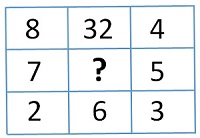a. 30

b. 35

c. 20

d. 25

17. Ramesh starts his journey by walking 2 kilometres towards North. Then he takes a right turn and walks 1 kilometre. Again takes a right turn and walks 2 kilometre. Now which direction is he facing?

a. East

b. West

c. South

d. North

18. Consider the given statement/s to be true and decide which of the given conclusions/assumptions can definitely be drawn from the given statement.

Statement: Irregularity is a cause for failure in exams. Some regular students fail in the examinations.

Conclusions:

I. All failed students are regular.

II. All successful students are not regular.

a. Only conclusion I follows

b. Only conclusion II follows

c. Both conclusion I and conclusion II follow

d. Neither conclusion I nor conclusion II follows

19. How many triangles are there in the given figure?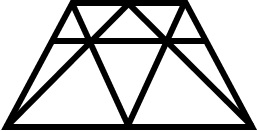a. 18

b. 19

c. 20

d. 21

20. Which one of the following figures represents the following relationship:

Birds, Parrots, Bats

a.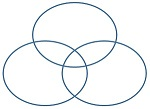b.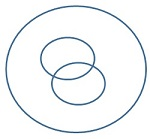c.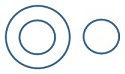d.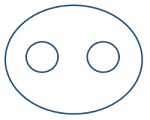21. Which answer figure will complete the pattern in the question figure?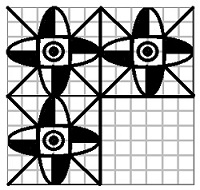a.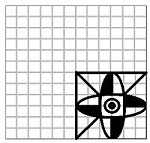b.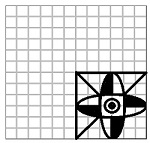c.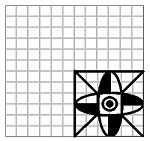d.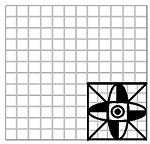22. From the given answer figures, select the one in which the question figure is hidden/embedded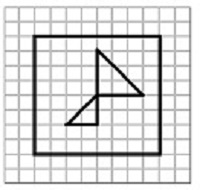a.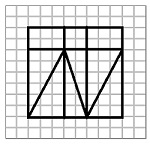b.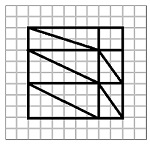c.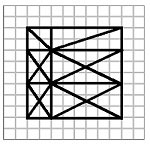d.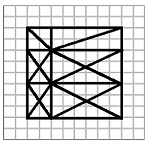23. A piece of paper is folded and cut as shown below in the question figures. From the given answer figures, indicate how it will appear when opened.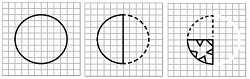a.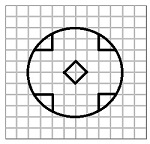b.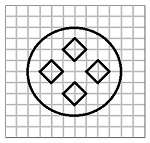c.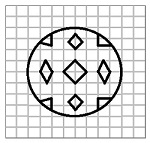d.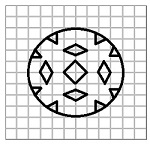24. If a mirror is placed on the line AB, then which of the answer figures is the right image of the given figure?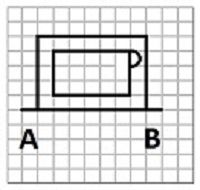a.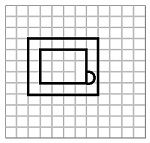b.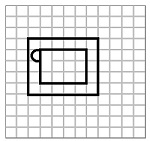c.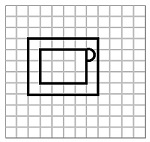d.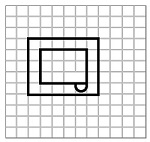25. In the question, a word is represented by only one set of numbers as given in any one of the alternatives. The sets of numbers given in the alternatives are represented by two classes of alphabets as in two matrices given below. The columns and rows of Matrix I are numbered from 0 to 4 and that of Matrix II are numbered from 5 to 9. A letter from these matrices can be represented first by its row and next by its column, e.g., ‘B’ can be represented by 00, 13 etc., and ‘A’ can be represented by 55, 69 etc.

Similarly you have to identify the set for the word ‘GIRL’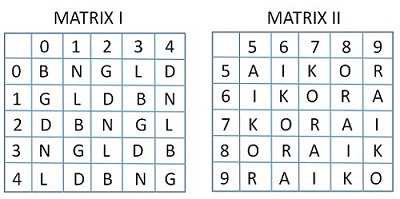a. 02, 56, 97, 24

b. 31, 79, 68, 42

c. 23, 97, 77, 11

d. 11, 88, 95, 23

All The Best To All The SSC CGL 2020 Aspirants !

Write a comment...Trending Articles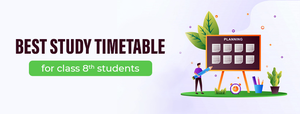By CBSE CLASS 8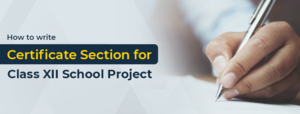By Edubabble LLP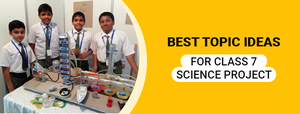By CBSE CLASS 7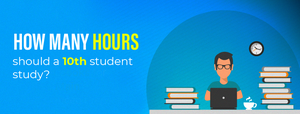By CBSE CLASS 10
Related PostsServicesNeed Some Help!Connect